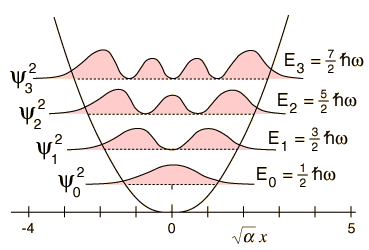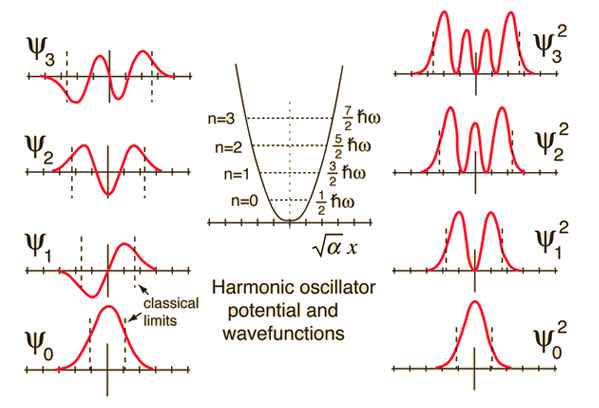# Probability Distributions for the Quantum Oscillator

The solution of the Schrodinger equation for the quantum harmonic oscillator gives the probability distributions for the quantum states of the oscillator.The solution gives the wavefunctions for the oscillator as well as the energy levels. The square of the wavefunction gives the probability of finding the oscillator at a particular value of x. Note that there is a finite probability that the oscillator will be found outside the "potential well" indicated by the smooth curve. This is forbidden in classical physics.Comparison of classical and quantum probabilities
Index

Schrodinger equation concepts

References
Beiser, Perspectives
Sec 8-7
Thornton & Rex
Sec 7-6

 HyperPhysics***** Quantum Physics R Nave
Go Back